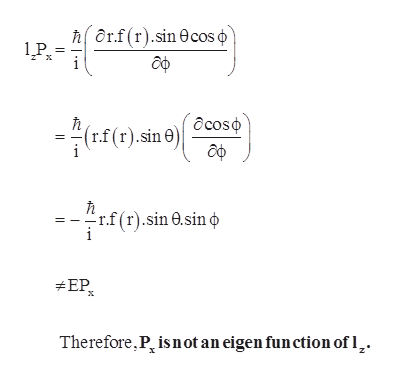Question
21 views

Determine whether p_x and p_y orbitals are eigenfunctions of I_z

check_circle

Step 1

An eigen value equation can be written in the following form,

(operator)(function) = (constant factor) x (same function)

Step 2

Whether Px is an eigen functions of Lz can be...help_outlineImage Transcriptionclosehorf (r).sin 0cos o 1P х ocosp (r.f (r).sin дф 'r.f(r).sin .sin #EP х is not an eigen fun ction of l Therefore, P | N fullscreen

### Want to see the full answer?

See Solution

#### Want to see this answer and more?

Solutions are written by subject experts who are available 24/7. Questions are typically answered within 1 hour.*

See Solution
*Response times may vary by subject and question.
Tagged in

### Chemistry